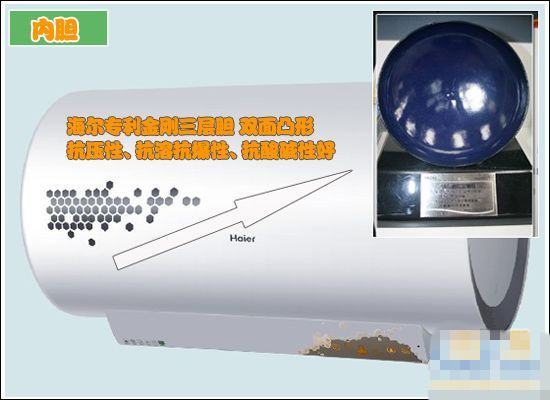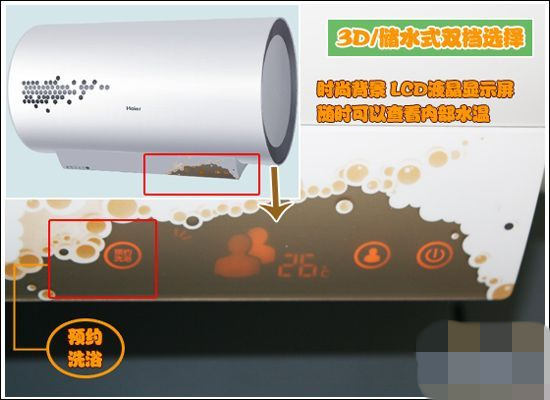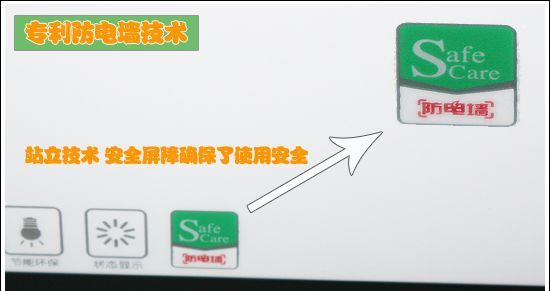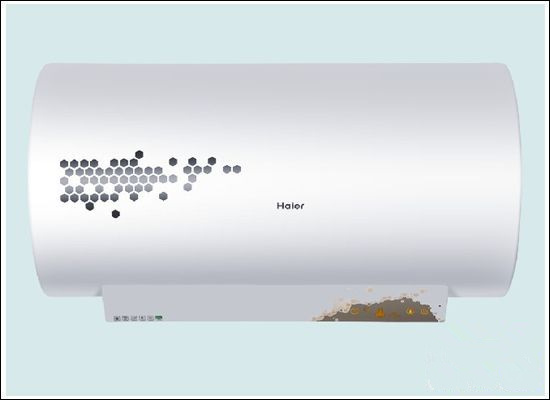|

# 3D速热技术 海尔新TT尊贵系列热水器测评

【导语】夏天来临，疲乏也更容易尾随而至，辛劳一天的人们回到家中冲一个热水澡，舒舒服服的休息一下想必是一件很爽的事情；那么，要想拥有这样的舒服与惬意，购买一款热水器就成了必要选择；可是在选购热水器的时候，作为外行的我们可能会苦恼与如何去选择，什么样的热水器才能既安全又实用呢？今天测评员就为大家介绍一款海尔升级版热水器——海尔新TT尊贵系列热水器ES80H-V1(E)，下面就一起去领略一下它的风采吧。

测评产品展示：测评详情：

1.海尔新TT尊贵系列热水器——经典外观设计从外观上看，海尔这款升级版的热水器依然是常见的圆柱造型，但是这个圆柱造型给人的感觉比普通版的圆柱热水器更美观，它摒弃了原始圆柱体的笨重，设计简洁大方，线条整体流畅，外壳色泽柔和亮丽，显得更加轻灵、更加时尚；热水器自身没有过多的装饰，而显示屏采用的是无边界的液晶显示屏，这样的设计在原有的造型中加入了时尚的元素，把浴室环境打造的更加柔和、典雅、舒适。

2.海尔新TT尊贵系列热水器--材质

一款好的热水器，它的材质的好坏是至关重要的，查看热水器的材质主要是看它的外壳和内胆；下面我们就具体来看一看海尔这款热水器的材质如何。

外壳材质：测评员手触热水器外壳，舒适中磨砂感；店员介绍，海尔这款热水器的外壳采用的是铝镁合金材质，表面涂有美国杜邦公司的伊丽莎白粉，这种材料经过酸洗、磷化、脱脂等多道工序严格把关，耐腐蚀性强，不易磨损，也不会随实用时间变化褪色，使用起来会持久颖新。

内胆：专利金刚三层胆热水器最重要的核心部位就是它的内胆，内胆的承压性关系到热水器的使用寿命和使用安全；鉴于中国水质复杂多样，国内热水器的使用也对它的内胆的抗压性提出了苛刻的要求；海尔这款热水器采用的是海尔专利金刚三层胆，采用国际先进对焊技术、搪瓷设备、搪瓷专用超细粉末，以世界先进工艺经过890度高温烧结而成，形成致密三层胆；抗溶、抗暴能力强；这种内胆经过了16万次承压检测，安全使用得到了保障。

因为条件限制，测评员无法拍摄到内胆温控器；据店员介绍，这款热水器的温控器是液体膨胀式温控器，深入内胆中部测温，相对于贴在内胆胆壁上的纽扣式温控器，反应更灵敏，测温也更准确。

3.海尔新TT尊贵系列热水器--功能技术从上图中我们可以看到，海尔新TT尊贵版热水器ES80H-V1(E)的主要优势功能有智能分人洗、3D动态加热、预约洗浴、节能环保、状态显示、防电墙等。智能分人洗就是可以选定一人洗、两人洗或者多人洗，系统会根据需要自动计算加热时间，这样还可以减少不必要的浪费；3D动态加热可以快速加热，即插即用；预约洗浴只需提前设好洗浴时间，热水器就会自动加热，在规定的时间内把热水烧好；四倍增容加热使得热水量比一般热水器量多，一定程度上节约了用电量；LCD液晶显示屏时尚美观且可以随时查看内部水温，使用更方便；而防电墙则保障了洗浴时的人身安全。

4.海尔新TT尊贵系列热水器--3D速热技术从上图的对比中我们就能看出，海尔3D速热技术加热的原理主要在它的高效聚焦环上；这种独特的高效聚焦环采用螺旋折叠结构，克服了以往加热中存在盲区的弊端，增加了加热体与水的接触面积，实现360度全方位加热无死角，加热均匀且速度也快，也使水在流出出水口时能被瞬间加热，实现开机即洗的功能，这个功能对悄然而至的夏天来说是非常实用的。

【常识】

热水气分类：热水器按照水热方式的不同分为：电热水器、燃气热水器和太阳能热水器三类。电热水器优点是安装简单，使用方便，不受天然气楼层气压差异的影响，越来越多的用户首选，缺点是它积极大占用空间；燃气热水器优点是热效率高、加热速度快、温度调节稳定、可多人连续使用，拥有一批固定的消费者，缺点是天然气成本逐渐提高；太阳能热水器属于第三代热水器，其节能概念已得到广泛认可，不但节能高效省钱，而且在使用时不会对环境造成污染，它的缺点是价格高，初期投资较大，且使用中易受天气影响。海尔采用特制配方的超长镁棒，大限度降低消耗，保护内胆；特制不锈钢内芯，可以确保镁棒不断裂，这样的镁棒更适合中国的水质，最大限度的确保内胆寿命更持久。

5.海尔新TT尊贵系列热水器--专利防电墙技术电热水器的实用中最大的隐患就是漏电造成人身伤害；为了确保自身产品实用的安全，保证人身不受伤害，海尔研发出自己专利的防电墙技术；这种防电墙技术相当于在热水器和人体之间加了一个很大的电阻，使人体有可能承受的电压低于12V，将其变为绝对安全电压，避免了安全隐患。

另外，针对中国家庭中普遍存在的环境带电等问题，海尔研发出了安全预警技术，一旦家中任何电器出现漏电导致地线带电，机身面板上的防电墙标志就会点亮，提醒您排除隐患，这一技术进一步保证了实用的安全性。

6.海尔新TT尊贵系列热水器--保温体统海尔经过繁复实验，寻找到更为节能的40度临界保温点，既免去了反复加热的浪费，又缩短了再次加热的时间，这样就实现了省时、省电的双量需求；而且它的保温层采用超细微孔发泡材料，发泡层采用的是与海尔冰箱相同的超细微孔发泡技术，精密控制发泡孔茎，降低了导热系数，是水温可以持久恒温；夏天即使不去加热，也可以冲一个不错的温水澡。

【小知识】热水器使用注意事项：

1、注意保持良好通风，不要在房间的供排气口上悬挂物品，以免影响空气流通。在每次使用前，都应检查安装热水器的房间窗户或排气扇是否打开，通风是否良好。

2、在使用过程中，需每隔一段时间对热水器进行安全检查。当发生漏烟、堵塞或者倒灌风时，请立即停止使用。

3、禁止在热水器周围放置易燃、易挥发性物品，禁止在排气口和供气口上放置毛巾、抹布等易燃品。

4、使用完后，检查燃气阀门是否关闭，经常检查供气管道是否完好。注意定期更换橡胶软管，请常用肥皂水在软管接驳处检查有无气泡出现，如有漏气，及时报修。

7.海尔新TT尊贵系列热水器--容量海尔新TT贵族版热水器有多个容量可供选择，如果您的洗漱间空间大，可以选择一款容量较大的热水器，最大容量的可达到100L；如果是精致的小户型，则可以选择小一点的热水器，最小容量可为50L；消费者可以根据自己的实际需求来选择；测评员今天为大家展示的这款容量为80L。

【测评总结】

海尔新TT尊贵系列热水器外观典雅美观，风格混搭，适合各种装修风格的居室实用；这款热水器在技术功能上也属一流，专利金刚三层胆保障了热水器的实用寿命，智能分人洗、3D速热技术、专利防电墙技术等都达到了实用、安全、节能的功效；测评员经过全方位的检测，综合比较，证明海尔这款热水器无论是外观还是实用性上都是很不错的。当然，好的东西需要的投入多，价格自然也会便宜，但与功能安全综合对比，海尔这款热水器算得上是性价比比较高的产品，消费者实用这样的产品也可以用的放心，稍高的价格应该是可以接受的。

`声明：本文由入驻焦点开放平台的作者撰写，除焦点官方账号外，观点仅代表作者本人，不代表焦点立场错误信息举报电话： 400-099-0099，邮箱：jubao@vip.sohu.com，或点此进行意见反馈，或点此进行举报投诉。`A B C D E F G H J K L M N P Q R S T W X Y Z
A - B - C - D - E
• A
• 鞍山
• 安庆
• 安阳
• 安顺
• 安康
• 澳门
• B
• 北京
• 保定
• 包头
• 巴彦淖尔
• 本溪
• 蚌埠
• 亳州
• 滨州
• 北海
• 百色
• 巴中
• 毕节
• 保山
• 宝鸡
• 白银
• 巴州
• C
• 承德
• 沧州
• 长治
• 赤峰
• 朝阳
• 长春
• 常州
• 滁州
• 池州
• 长沙
• 常德
• 郴州
• 潮州
• 崇左
• 重庆
• 成都
• 楚雄
• 昌都
• 慈溪
• 常熟
• D
• 大同
• 大连
• 丹东
• 大庆
• 东营
• 德州
• 东莞
• 德阳
• 达州
• 大理
• 德宏
• 定西
• 儋州
• 东平
• E
• 鄂尔多斯
• 鄂州
• 恩施
F - G - H - I - J
• F
• 抚顺
• 阜新
• 阜阳
• 福州
• 抚州
• 佛山
• 防城港
• G
• 赣州
• 广州
• 桂林
• 贵港
• 广元
• 广安
• 贵阳
• 固原
• H
• 邯郸
• 衡水
• 呼和浩特
• 呼伦贝尔
• 葫芦岛
• 哈尔滨
• 黑河
• 淮安
• 杭州
• 湖州
• 合肥
• 淮南
• 淮北
• 黄山
• 菏泽
• 鹤壁
• 黄石
• 黄冈
• 衡阳
• 怀化
• 惠州
• 河源
• 贺州
• 河池
• 海口
• 红河
• 汉中
• 海东
• 怀来
• I
• J
• 晋中
• 锦州
• 吉林
• 鸡西
• 佳木斯
• 嘉兴
• 金华
• 景德镇
• 九江
• 吉安
• 济南
• 济宁
• 焦作
• 荆门
• 荆州
• 江门
• 揭阳
• 金昌
• 酒泉
• 嘉峪关
K - L - M - N - P
• K
• 开封
• 昆明
• 昆山
• L
• 廊坊
• 临汾
• 辽阳
• 连云港
• 丽水
• 六安
• 龙岩
• 莱芜
• 临沂
• 聊城
• 洛阳
• 漯河
• 娄底
• 柳州
• 来宾
• 泸州
• 乐山
• 六盘水
• 丽江
• 临沧
• 拉萨
• 林芝
• 兰州
• 陇南
• M
• 牡丹江
• 马鞍山
• 茂名
• 梅州
• 绵阳
• 眉山
• N
• 南京
• 南通
• 宁波
• 南平
• 宁德
• 南昌
• 南阳
• 南宁
• 内江
• 南充
• P
• 盘锦
• 莆田
• 平顶山
• 濮阳
• 攀枝花
• 普洱
• 平凉
Q - R - S - T - W
• Q
• 秦皇岛
• 齐齐哈尔
• 衢州
• 泉州
• 青岛
• 清远
• 钦州
• 黔南
• 曲靖
• 庆阳
• R
• 日照
• 日喀则
• S
• 石家庄
• 沈阳
• 双鸭山
• 绥化
• 上海
• 苏州
• 宿迁
• 绍兴
• 宿州
• 三明
• 上饶
• 三门峡
• 商丘
• 十堰
• 随州
• 邵阳
• 韶关
• 深圳
• 汕头
• 汕尾
• 三亚
• 三沙
• 遂宁
• 山南
• 商洛
• 石嘴山
• T
• 天津
• 唐山
• 太原
• 通辽
• 铁岭
• 泰州
• 台州
• 铜陵
• 泰安
• 铜仁
• 铜川
• 天水
• 天门
• W
• 乌海
• 乌兰察布
• 无锡
• 温州
• 芜湖
• 潍坊
• 威海
• 武汉
• 梧州
• 渭南
• 武威
• 吴忠
• 乌鲁木齐
X - Y - Z
• X
• 邢台
• 徐州
• 宣城
• 厦门
• 新乡
• 许昌
• 信阳
• 襄阳
• 孝感
• 咸宁
• 湘潭
• 湘西
• 西双版纳
• 西安
• 咸阳
• 西宁
• 仙桃
• 西昌
• Y
• 运城
• 营口
• 盐城
• 扬州
• 鹰潭
• 宜春
• 烟台
• 宜昌
• 岳阳
• 益阳
• 永州
• 阳江
• 云浮
• 玉林
• 宜宾
• 雅安
• 玉溪
• 延安
• 榆林
• 银川
• Z
• 张家口
• 镇江
• 舟山
• 漳州
• 淄博
• 枣庄
• 郑州
• 周口
• 驻马店
• 株洲
• 张家界
• 珠海
• 湛江
• 肇庆
• 中山
• 自贡
• 资阳
• 遵义
• 昭通
• 张掖
• 中卫

1室1厅1厨1卫1阳台

1
2
3
4
5

0
1
2

1

1

0
1
2
3报名成功，资料已提交审核A B C D E F G H J K L M N P Q R S T W X Y Z
A - B - C - D - E
• A
• 鞍山
• 安庆
• 安阳
• 安顺
• 安康
• 澳门
• B
• 北京
• 保定
• 包头
• 巴彦淖尔
• 本溪
• 蚌埠
• 亳州
• 滨州
• 北海
• 百色
• 巴中
• 毕节
• 保山
• 宝鸡
• 白银
• 巴州
• C
• 承德
• 沧州
• 长治
• 赤峰
• 朝阳
• 长春
• 常州
• 滁州
• 池州
• 长沙
• 常德
• 郴州
• 潮州
• 崇左
• 重庆
• 成都
• 楚雄
• 昌都
• 慈溪
• 常熟
• D
• 大同
• 大连
• 丹东
• 大庆
• 东营
• 德州
• 东莞
• 德阳
• 达州
• 大理
• 德宏
• 定西
• 儋州
• 东平
• E
• 鄂尔多斯
• 鄂州
• 恩施
F - G - H - I - J
• F
• 抚顺
• 阜新
• 阜阳
• 福州
• 抚州
• 佛山
• 防城港
• G
• 赣州
• 广州
• 桂林
• 贵港
• 广元
• 广安
• 贵阳
• 固原
• H
• 邯郸
• 衡水
• 呼和浩特
• 呼伦贝尔
• 葫芦岛
• 哈尔滨
• 黑河
• 淮安
• 杭州
• 湖州
• 合肥
• 淮南
• 淮北
• 黄山
• 菏泽
• 鹤壁
• 黄石
• 黄冈
• 衡阳
• 怀化
• 惠州
• 河源
• 贺州
• 河池
• 海口
• 红河
• 汉中
• 海东
• 怀来
• I
• J
• 晋中
• 锦州
• 吉林
• 鸡西
• 佳木斯
• 嘉兴
• 金华
• 景德镇
• 九江
• 吉安
• 济南
• 济宁
• 焦作
• 荆门
• 荆州
• 江门
• 揭阳
• 金昌
• 酒泉
• 嘉峪关
K - L - M - N - P
• K
• 开封
• 昆明
• 昆山
• L
• 廊坊
• 临汾
• 辽阳
• 连云港
• 丽水
• 六安
• 龙岩
• 莱芜
• 临沂
• 聊城
• 洛阳
• 漯河
• 娄底
• 柳州
• 来宾
• 泸州
• 乐山
• 六盘水
• 丽江
• 临沧
• 拉萨
• 林芝
• 兰州
• 陇南
• M
• 牡丹江
• 马鞍山
• 茂名
• 梅州
• 绵阳
• 眉山
• N
• 南京
• 南通
• 宁波
• 南平
• 宁德
• 南昌
• 南阳
• 南宁
• 内江
• 南充
• P
• 盘锦
• 莆田
• 平顶山
• 濮阳
• 攀枝花
• 普洱
• 平凉
Q - R - S - T - W
• Q
• 秦皇岛
• 齐齐哈尔
• 衢州
• 泉州
• 青岛
• 清远
• 钦州
• 黔南
• 曲靖
• 庆阳
• R
• 日照
• 日喀则
• S
• 石家庄
• 沈阳
• 双鸭山
• 绥化
• 上海
• 苏州
• 宿迁
• 绍兴
• 宿州
• 三明
• 上饶
• 三门峡
• 商丘
• 十堰
• 随州
• 邵阳
• 韶关
• 深圳
• 汕头
• 汕尾
• 三亚
• 三沙
• 遂宁
• 山南
• 商洛
• 石嘴山
• T
• 天津
• 唐山
• 太原
• 通辽
• 铁岭
• 泰州
• 台州
• 铜陵
• 泰安
• 铜仁
• 铜川
• 天水
• 天门
• W
• 乌海
• 乌兰察布
• 无锡
• 温州
• 芜湖
• 潍坊
• 威海
• 武汉
• 梧州
• 渭南
• 武威
• 吴忠
• 乌鲁木齐
X - Y - Z
• X
• 邢台
• 徐州
• 宣城
• 厦门
• 新乡
• 许昌
• 信阳
• 襄阳
• 孝感
• 咸宁
• 湘潭
• 湘西
• 西双版纳
• 西安
• 咸阳
• 西宁
• 仙桃
• 西昌
• Y
• 运城
• 营口
• 盐城
• 扬州
• 鹰潭
• 宜春
• 烟台
• 宜昌
• 岳阳
• 益阳
• 永州
• 阳江
• 云浮
• 玉林
• 宜宾
• 雅安
• 玉溪
• 延安
• 榆林
• 银川
• Z
• 张家口
• 镇江
• 舟山
• 漳州
• 淄博
• 枣庄
• 郑州
• 周口
• 驻马店
• 株洲
• 张家界
• 珠海
• 湛江
• 肇庆
• 中山
• 自贡
• 资阳
• 遵义
• 昭通
• 张掖
• 中卫• 手机• 分享
• 设计
免费设计
• 计算器
装修计算器
• 入驻
合作入驻
• 联系
联系我们
• 置顶
返回顶部